## Friday, April 24, 2009

### Pascal's triangle: a neat proof

There is an interesting question about how the terms in Pascal's triangle grow. All the terms in a row obviously grow (except the 1s at the extreme left- and right-hand sides of the triangle), but the rows' totals obviously grow, too. Do any of the terms in a row converge, as a percentage of the total of the row?

The most interesting example is the middle term in a row, e.g.:

1
1 1
1 2 1

the 1 and 2 are middle terms for their respective rows. The middle term is always the largest term and grows quickly. So, which grows faster: the row total or its middle term, or is there a convergence? A few examples illustrate what happens:

The 0th row -- 100%
The 2nd row -- 50%The numerator is the formula for the middle term (see the binomial distribution); the denominator is the row's total. The largest row that my TI-84 calculator could handle before overflowing is the 332nd. As the examples show, the denominator is "winning" -- the middle term as a percentage of the total is steadily decreasing. Pretty amazing considering just how big the middle term is; for the 332nd row, the numerator is 3.8E98!

Can we write a proof of this? Certainly. The first step is to re-write the numerator. Given that the combination is really a simplification ofso, our specific numerator can be re-written as: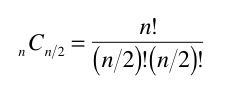To re-write the limit for our whole expression, the middle term divided by the row total, would look like this: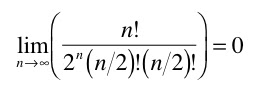Now the clever step. Let's re-organize that n factorial in the numerator and expand the terms in the denominator:All the n factorial terms are there; they just been re-organized, with the even terms on one side and the odd terms on the other. It should make sense that the terms in the numerator "sit" perfectly in the "seats" provided by the denominator: there are n terms in the numerator, and there are two groups of n/2 terms in the denominator.

The proof is basically done. One simple re-write makes the final conclusion clear: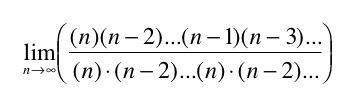Each fraction is now on its own. The first set of fractions are all 1s. Every other fraction must be less than 1. An infinite multiplication of fractions less than or equal to 1 must converge to 0. So, in an infinite Pascal's triangle, the middle term, although it increases without bound, is 0% of the row's total.

---

The above paragraph is incorrect. A lot more work must be done to show that the product of the remaining fractionsgoes to zero. I like explanations that just make intuitive sense. So, the first term is 0.5. I noticed that the product of the next four terms (terms two to five) is slightly less than 0.5. A little cancelation helps make why clear:Thus:And this continues. The product of the next sixteen terms (terms six to twenty-one) is also less than 0.5. Here are the terms: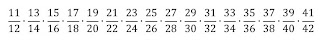A litte cancelation starts the process: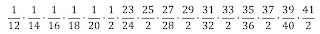and reorganizing the denominators makes the result clear:Each pair of terms is slightly less than one. Each pair of terms can be written in the form: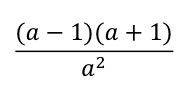or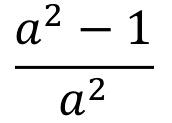The product of the next 64 terms is also less than 0.5. (The number of terms is based on powers of 4.) So, the whole product can be rewritten as 0.5^infinity... that definitely goes to zero.

1."An infinite multiplication of fractions less than or equal to 1 must converge to 0."

This is a false statement

2.For n = 1 to inf, The product of (4n^2-1)/(4n^2) = 2/pi

where (4n^2-1)/(4n^2)<1 for all natural numbers, n

3.Hmm, neat. You're right.

4.I read your proof a while back and your statement "seemed" right to me too. I was hesitant to accept it at face value, but I couldn't prove it one way or another. Then today I saw the mentioned product on another site and remembered this post.

I still "believe" your final conclusion is true but the proof isn't htere just yet.

Pascal's Traingle intrigues me and I'm interested if you had a use in mind for your conclusion.

5.With regard to:

For n = 1 to inf, The product of (4n^2-1)/(4n^2) = 2/pi

where (4n^2-1)/(4n^2)<1 for all natural numbers, n

I just wrote a little program to check this, and it seemed to be false. Here's a code fragment:

product = 1;
for(i=0; i< k; i++){

product = product * (4 * n*n - 1) / (4 * n * n);

}

For large values of k, the product approaches zero.

6.Never mind. It's true. The correct code is:

product=1;
for(i=1; i< n; i++){

product = product * (4 * i*i - 1) / (4 * i * i);

}

7.8.Consider this approach: rather than comparing the center term to the sum of the row, compare it to the two adjacent terms:

lim n-> inf {C(n,n/2)/[C(n,n/2-1)+C(n/n/2+1)]}
= lim n->inf {C(n/n/2)/[2*C(n,n/2-1)}
It's easily shown that this goes to 1/2

Do the same for the next two terms out:
lim n->inf {C(n/n/2)/[2*C(n,n/2-2)}
lim n-> inf {C(n,n/2)/[C(n,n/2-1)+C(n/n/2+1)]}

This continues as you choose the next two terms. Since the middle term approaches 1/2 the adjacent terms and appoaches 1/2 the next adjacent terms or 1/4 the 4 closest terms, I'm sure with some effort, it can be shown that the middle term approaches 1/(2^n) the sum of the 2n "closest terms" so the fraction of the center term to the sum of the row can be shown to be < 1/(2^n) for any n we choose.

Perhaps you can come up with a formalized version of this. It's not a nice, neat proof, but it can be done. Perhaps by contradiction assuming that the center term divided by the sum of the row is > 1/2^n for some arbitrary n and disproving this. I don't know if this helps.

9.Actually a delta-epsilon proof on the limit works fine since for 0 < epsilon, you can find N such than n>N -> 0 < 1/(2^n) < epsilon.

10.Another possible solution:

Let L(n) = Product[i=1 to n/2| (2i-1)/(2i)] for even n
The last limit in your proof is lim[n->inf | L(n)
It can be shown simply enough:
0 < L(n) <= 1/sqrt(n)

So by the Squeeze (Sandwich) Theorem, your limit goes to zero.

11.Hi John,

I'm sorry it took me so long to respond; it's a busy time at school.

I'm not sure I understand either of your proposed methods correctly.

For the first, you write "rather than comparing the center term to the sum of the row, compare it to the two adjacent terms. It's easily shown that this goes to 1/2." I must misunderstand what you're suggesting -- for example, 200 C 101 divided by 200 C 100 (next-to-middle / middle term) is .99 --the ratio of the middle terms to the next terms approaches 1, not 1/2.

For the second proposal, I agree that the critical terms in the convergence is product [i=1 to inf.] of (2i-1)/2i, but I think L(n) is greater than 1/sqrt(n). For example, n=5, so 9/10 > 1/sqrt(5).

12.Sorry, my notation is terrible. I don't know how to get an equation editor to output anything that I can paste into this comment.

For the first, your comparing it to the sum of the two adjacent terms or:
200C100 / (200C99 + 200C101) which goes to 1/2
Doing the same with the next two terms, 200C98 + 200C102 yields the same result as you take it to infinity and so on.

For the second L(N) is the product from i = 1 to n/2 of the values (2i-1)/(2i). L(N) is defined for even n.
For n= 6, L(n) = 1/2 * 3/4 * 5/6 = 15/48
and 15/48 < 1/sqrt(6).

By the way, how are you getting your equations into the post. Are you converting them to jpg's or bmp's?

13.All of the N's in the previous post should be lower case

14.What field of mathematics is this sort of maths classified under?

I am interested in this type of maths.

15.I would put this under the topic "infinite products."

16.What I mean is: does this type of maths fall under a subject like number theory? If not, what other broad range in maths is it?

Thanks for responding.

17.Yes, number theory would be the broad topic, I guess.

18.It occurred to me, after all these years, that there is an important, obvious connection to the Normal probability density function. The binomial distribution, which is modeled by Pascal's triangle, gives rise to the Normal distribution as n goes to infinity. So of course the probability of any individual outcome goes to zero; that is how the Normal distribution works. It only makes sense to speak of the probability of a specific range of outcomes in a Normal distribution.